## What is Net Book Value of Assets?

Net book value, also known as net asset value, is the value at which a company reports an asset on its balance sheet. It is calculated as the original cost of an asset less accumulated depreciation, accumulated amortization, accumulated depletion or accumulated impairment.

## Key Learning Points

• Net book value or net asset value is the value an asset is reported in a company’s set of accounts.
• Net book value is calculated as the asset’s original cost less accumulated depreciation, depletion, and impairment.
• The balance sheet is a financial statement that reports the financial position of a company at a point in time with all assets being reported at their net book value (NBV).
• The original cost of an asset is the total cost incurred by a company to purchase and deliver an asset for its intended use.
• The net book value of an asset is rarely equal to its market value, instead, it shows the value of the asset after deducting accumulated depreciation which is based on prudent accounting principles.

## Formula

The formula to calculate net book value is: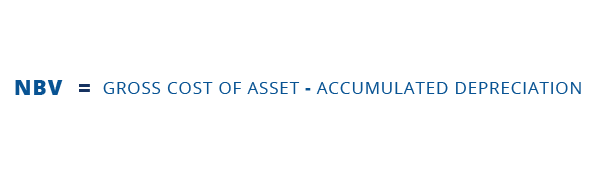Let’s start by calculating the original cost of an asset.

The original cost of an asset includes the original cost of acquisition plus any costs associated with the delivery, and intended use of an asset to the purchase price. As an example, the original cost of an asset can include the purchase price, delivery fees, setup costs and customs duties.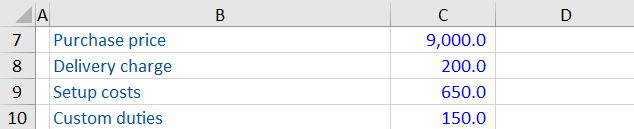### Deductions

As mentioned above, there are several expenses you must deduct from the original cost of an asset to get the net book value. Let’s look at depreciation, amortization and depletion. These expenses are a ratable charge over the asset’s useful life. This means the net book value of an asset should decrease at a predictable rate throughout the asset’s life.

An impairment is an extraordinary loss in the value of an asset. Businesses must write down the value of their asset if the recoverability of the net book value is in doubt.

### Example:

Company XYZ acquired an asset for \$10,000 and uses the straight-line method of depreciation. It expects the machine to have a useful life of 10 years.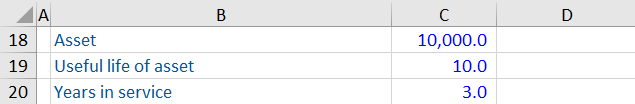To calculate annual depreciation: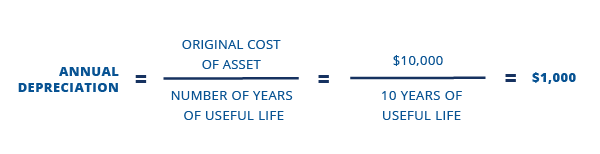The calculation becomes the following: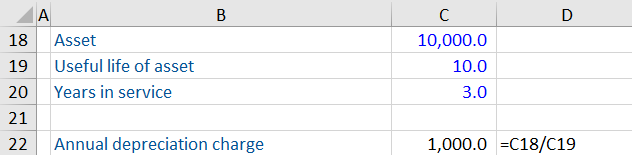If Company XYZ had the asset for 3 years, then the accumulated depreciation would be 3,000.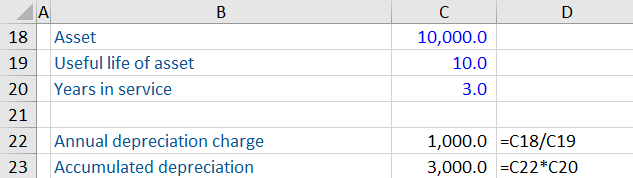### Net Book Value Calculation

We mentioned above that you deduct accumulated depreciation from the original cost of an asset to get the net book value.

If we use the figures from above, it works out as follows: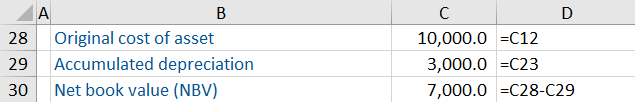## Why is Net Book Value Important?

The net book value of a company is not the same as the market value of a company, since the book values of the assets and liabilities are not the same as the market values of all the assets and liabilities. However, net book value does provide an important function for users of accounts since it is based on prudent principles, and can sometimes be used to indicate the minimum value (or floor value) that the company is worth.